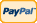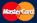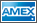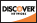Online Store Software Catalog and Price Product Registration Services News About Us Contact Us My Account Español
•Electronics, electrical engineering
•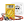Engineering physics
•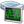Generators
•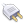Interfaces
•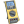Multimeters
•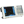Oscilloscopes
•Persona
•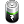Power supplies
•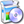Software
•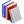Standards
•Thesaurus
•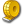Units of measurement
Filter by first letter
A
B
C
D
E
F
G
H
I
J
K
L
M
N
O
P
Q
R
S
T
U
V
W
X
Y
Z
+
-

# Crms (Cycrms)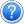Search:

A type of automatic measurements. The true Root Mean Square voltage over the first cycle in the waveform.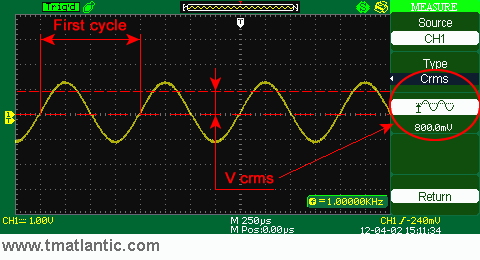Cycrms (or Crms) - RMS (root-mean-square) voltage is useful when dealing in period signals; calculation of RMS voltage involves integration over one period and division by the length of the period. It is chiefly used with sine wave functions, and many times when a sine wave is specified its amplitude will be given as an RMS voltage.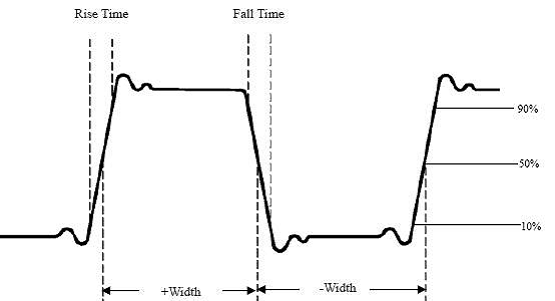• Pk-Pk (Vp): amplitude (peak-to-peak) voltage Max (Ma): the maximum voltage
• Min (Mi): the minimum voltage
• Vtop (Vt): rectangular pulse voltage peaks
• Vbase (Vb): base voltage square wave
• Vamp (Va): voltage amplitude (Vtop - Vbase)
• Mean (V): the average value of the voltage
• Cycrms (Vk): rms voltage over one period
• (Vmax-Vtop)/Vamp->Overshoot (Os): Emission measurements at the top of a percentage of the amplitude of the square wave (Vmax-Vtop) / Vamp
• (Vmin-Vbase)/Vamp->Preshoot (Ps): Emission measurements at the base as a percentage of the amplitude of the rectangular pulse (Vmin-Vbase) / Vamp

Back to the list

Units Converter
• How it Works
• Company News
• Industry News & Events
• Measurement Fun Facts